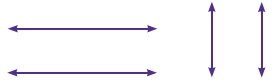Home | | Maths 6th Std | Exercise 4.2

# Exercise 4.2

6th Maths : Term 2 Unit 4 : Geometry : Exercise 4.2 : Text Book Back Exercises Questions with Answers, Solution

Exercise 4.2

1. Draw a line segment AB = 7 cm and mark a point P on it. Draw a line perpendicular to the given line segment at P.Step 1 : Draw a line AB = 7 cm and take a point P on the line

Step 2 : Place the set square on the line in such a way that the vertex which forms right angle coincides with P and one arm of the right angle coincides with the line AB.

Step 3 : Draw a line PQ through P along the other arm of the right angle at the set square.

Step 4 : The line PQ is perpendicular to the line AB at P.

That is PQ AB.

APQ = BPQ = 90°

2. Draw a line segment LM = 6.5 cm and take a point P not lying on it. Using a set square construct a line perpendicular to LM through P.Step 1 : Draw a line LM = 6.5 cm. Take a point P above the line LM.

Step 2 : Place one of the arms of the right angle of a set square along the line LM and the other arm of its right angle touches the point P.

Step 3 : Draw a line through the point P meeting LM at Q.

Step 4 : The line PQ is perpendicular to the line LM at Q.

That is PQ LM.

3. Find the distance between the given lines using a set square at two different points on each of the pairs of lines and check whether they are parallel.Yes.

They are parallel.

4. Draw a line segment measuring 7.8 cm. Mark a point B above it at a distance of 5 cm.

Through B draw a line parallel to the given line segment.Step 1 : Draw a line segment xy = 7.8 cm and mark a point A on the line.

Step 2 : Place the set square in such away that the vertex of the right angle coincides with A and one of the edges of right angle lies along XY mark the point B such that AB = 5 cm.

Step 3 : Place the set square under the line XY and fix the scale touching the set square.

Step 4 : Hold the scale firmly and slide the set square along the edge of the scale until the other edge touches the point B. Draw a line BC through B.

Step 5 : The line BC is parallel to XY. That is, BC || XY.

5. Draw a line and mark a point R below it at a distance of 5.4 cm Through R draw a line parallel to the given line.Step 1 : Using a scale draw a line AB and mark a point Con the line.

Step 2 : Place the set square upside down such that the vertex of the right angle coincides with C and one of the edges of right angle lies along AB. Mark a point R below AB such that CR = 5.4 cm

Step 3 : Place the setsquare below AB and fix the scale touching the set square.

Step 4 : Hold the scale firmly and slide the set square down until the other edge touches the point R. Draw a line RS through R.

Step 5 : The line RS is parallel to AB. That is RS || AB.

Tags : Questions with Answers, Solution | Geometry | Term 2 Chapter 4 | 6th Maths , 6th Maths : Term 2 Unit 4 : Geometry
Study Material, Lecturing Notes, Assignment, Reference, Wiki description explanation, brief detail
6th Maths : Term 2 Unit 4 : Geometry : Exercise 4.2 | Questions with Answers, Solution | Geometry | Term 2 Chapter 4 | 6th Maths